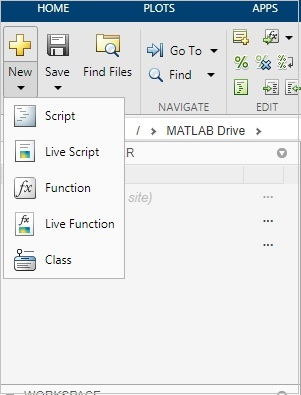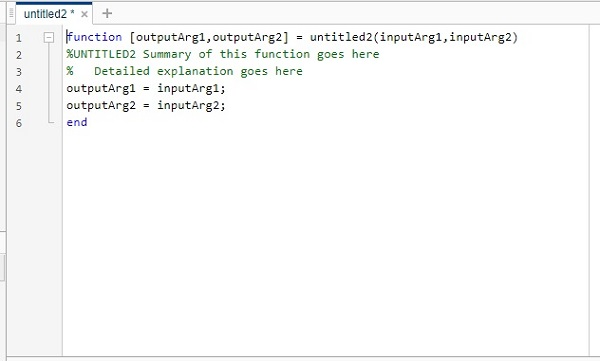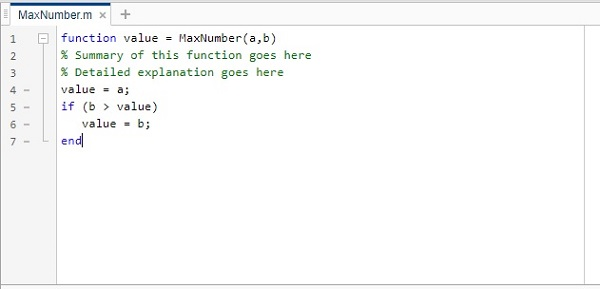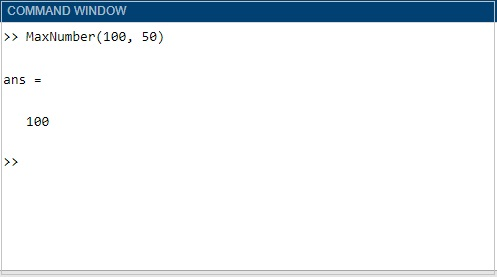# Matlab M-Files - Functions

#### Data Preprocessing for Machine Learning using MATLAB

30 Lectures 4 hours

#### Image Processing Toolbox in MATLAB

17 Lectures 3 hours

#### Matlab - The Complete Course

Best Seller

37 Lectures 5 hours

A function is a group of statements that together perform a task. In MATLAB, functions are defined in separate files. The name of the file and name of the function should be thesame.

Functions operate on variables within their own workspace, which is also called the local workspace. These functions separate the variables from the workspace which you access at the MATLAB command prompt. This is called the base workspace.

Functions can accept more than one input arguments and may return more than one output arguments.

The syntax of a function statement is as follows −

```function [out1,out2, ..., outN] = myfun(in1,in2,in3, ..., inN)
```

Here out1, out2...outN are output variables. It can be a single variable or comma separated. The variables in1, in2, in3...inN are input variables which can be single variable or comma separated ones. The function in MATLAB starts with the keyword function as shown in the syntax.

While naming your m-file, you should take care that the file name and the function name has to match. You cannot use the name of any built-in function available in MATLAB.

Let us now create a simple function and save it as .m file and run it. In MATLAB, the IDE allows you to select what type of file you want to create as shown below −Click on the Function, and it will open a new file as shown below −Now you can update the output variables, the function name and the input variables in the above untitled file and save the file with the same name as the function name.The name of our function is MaxNumber() and it gives the maximum number from the input value passed.

Now let us run the function to get the output. You can call the function by using MaxNumber(100,50).